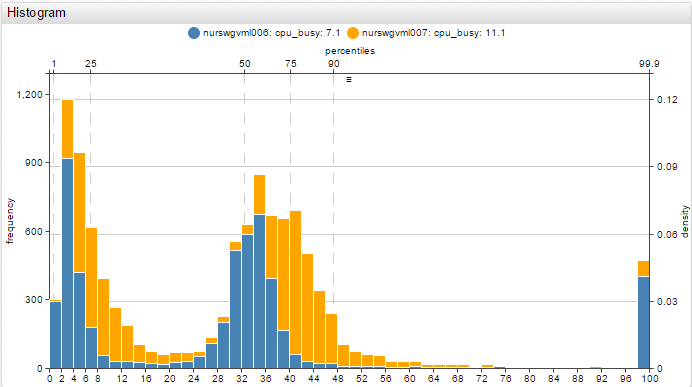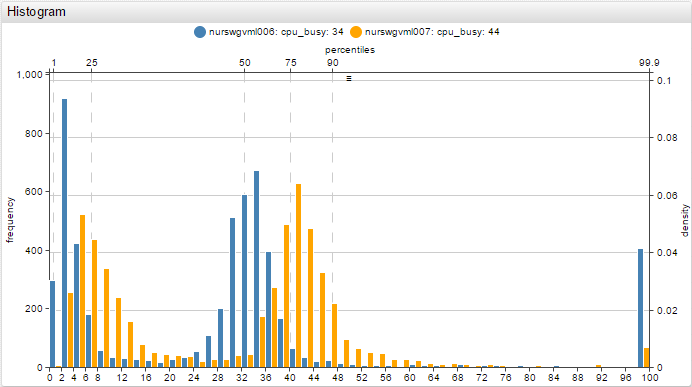# Histogram Chart

Histogram visualizes the distribution of data.

StackedChart Lab: View

Multiple SeriesChart Lab: View

#### [widget] settings

Inherited settings from Generic Widget: type, title, tooltip, left-units, top-units, width-units, height-units, timespan, path, url, alert-style, alert-expression, style, class, format, min-range, max-range, min-range-force, max-range-force, legend-position, rotate-ticks, axis-title, axis-title-right.

NameExampleDescriptionChart Lab
mode`mode = stack`If `mode = stack` and multiple series are configured, data is merged from all series into one data array.
If mode is not set to stack, each series of the histogram will be displayed separately.
View
bar-count`bar-count = 20`Number of bars (distributions).
Default value: 50
Bar count can also be controlled from the mouseover menu in the upper right-hand corner of the widget.
View
left-axis`left-axis = frequency`Values displayed on the left-axis.
Possible values: none, density, fraction, frequency
Default value: frequency
`density` – scales the height of the bars so that the sum of their areas equals 1.
`fractions` – scales the height of the bars so that the sum of their heights equals 1.
`frequency` – scales the height of the bars so that each bar’s height is equal to the number of observations in the series. Thus the sum of the heights is equal to the total number of observations.
View
right-axis`right-axis = density`Values displayed on the right-axis.
Possible values: none, density, fraction, frequency
Default value: none
`density` – scales the height of the bars so that the sum of their areas equals 1.
`fractions` – scales the height of the bars so that the sum of their heights equals 1.
`frequency` – scales the height of the bars so that each bar’s height is equal to the number of observations in the series. Thus the sum of the heights is equal to the total number of observations.
View
top-axis`top-axis = percentiles`Values displayed on the top-axis.
Possible values: none, percentiles, values
Default value: none
View
bottom-axis`bottom-axis = percentiles`Values displayed on the bottom-axis.
Possible values: none, percentiles, values
Default value: values
View
percentiles`percentiles = 1, 10, 50, 90, 99.9`Percentiles that will displayed when `top-axis = percentiles`.
Multiple percentiles must be separated with commas.
View
vertical-grid`vertical-grid = percentiles`Display a vertical line corresponding to each percentile on the top-axis.
Possible values: none, percentiles
Default value: none
View
horizontal-grid`horizontal-grid = frequency`Display a horizontal line corresponding to each value on the right- or left-axis.
Possible values: none, density, fractions, frequency
Default value: frequency
View
min-range
max-range
`min-range = 20`
`max-range = 90`
Set the minimum and maximum range of the x-axis.
If value is outside the set max/min then it is added to the max/min bucket in the visible range.
View
min-range-force
max-range-force
`min-range-force = 20`
`max-range-force = 90`
Set the strict minimum and maximum range of the x-axis.View
range-offset`range-offset = 50`Adds an offset to the left and right of the dataset, set in pixels.
Off by default.
View

#### [series] settings

Inherited settings from Generic Widget [series]: entity, metric, type, interval, style, color, label, tooltip, display, hidden, refresh-interval, retry-refresh-interval, error-refresh-interval

#### Particular series can be filtered using the `display` setting.

Filter series based on time lag – View Chart Lab
Filter series that are not in the top-2 by last value – View Chart Lab
Filter series if last value is less than 10 – View Chart Lab

#### Configuration Example:

```[widget]   time-span = 1 day type = histogram min-range = 0 max-range = 100 top-axis = percentiles   percentiles = 1, 25, 50, 75, 90, 99.9   [series] entity = nurswgvml007 metric = cpu_busy```